# transient analysis produce results same to static analysis

hi everyone

i have a problem in transient analysis as the output displacement is similar to static analysis and different from hand calculations

the structure i am analyzing is a cantilever column with point mass at the top with applied transient load at the top of the column and damping ratio of 10%

for the time step i used 2 initial steps with time integration off from 0 to .0001 & from .0001 to .0002 in order to have zero velocity initial condition and then applied load from the next steps with time integration on

here are some photos describing the problem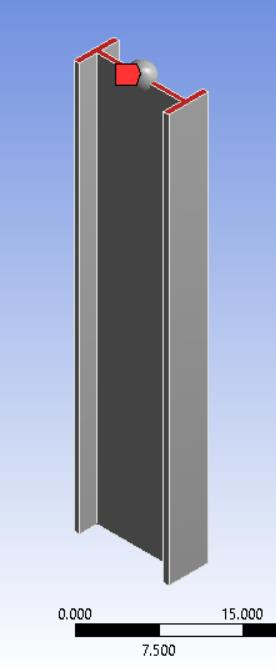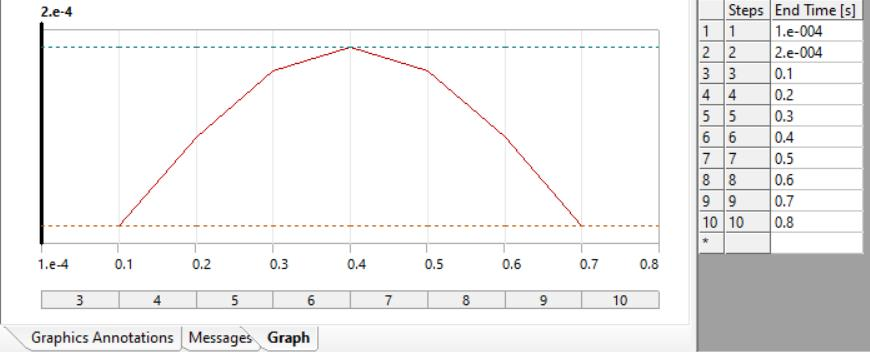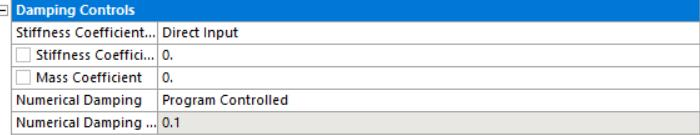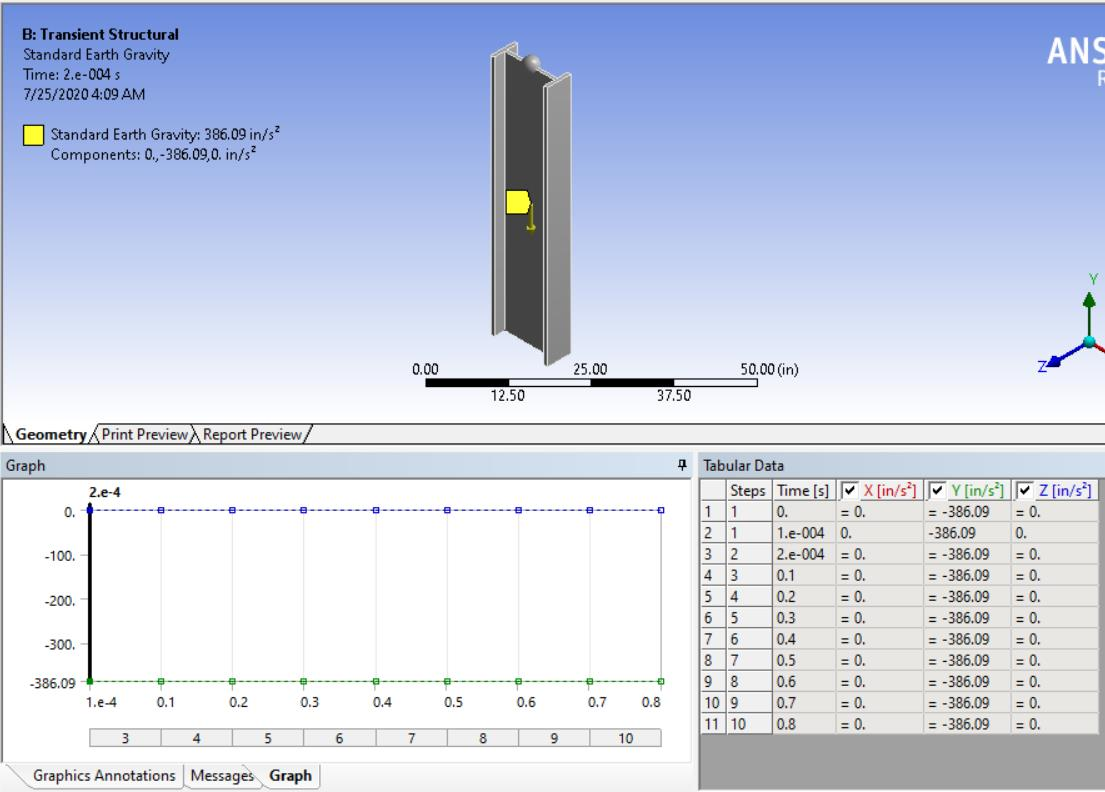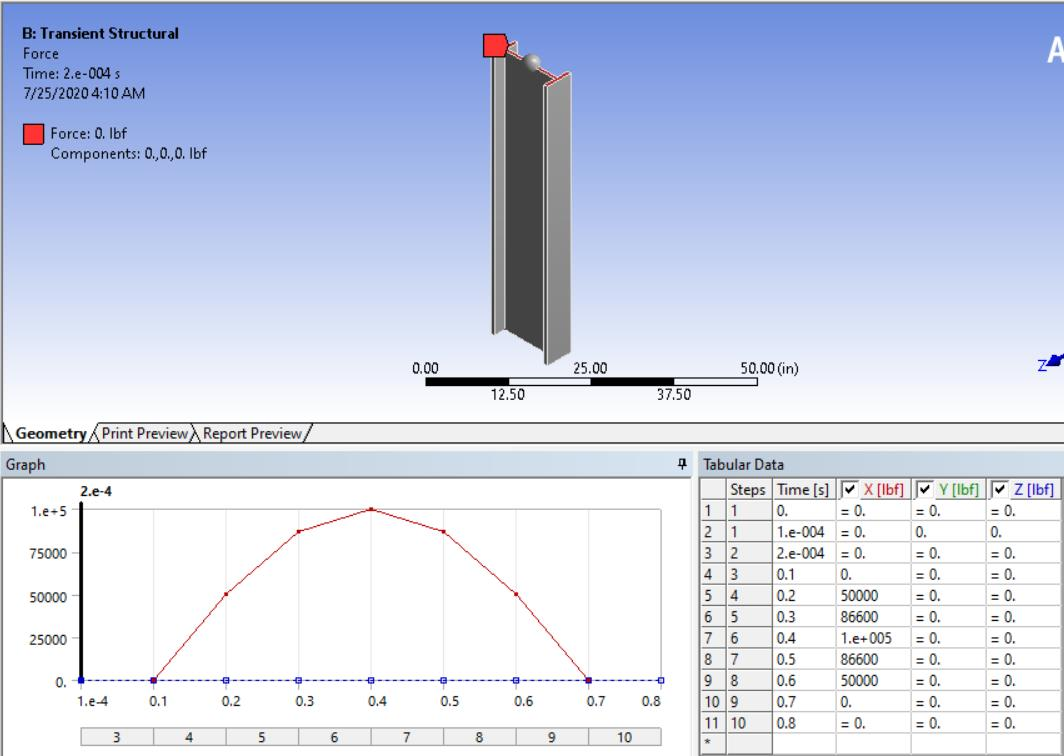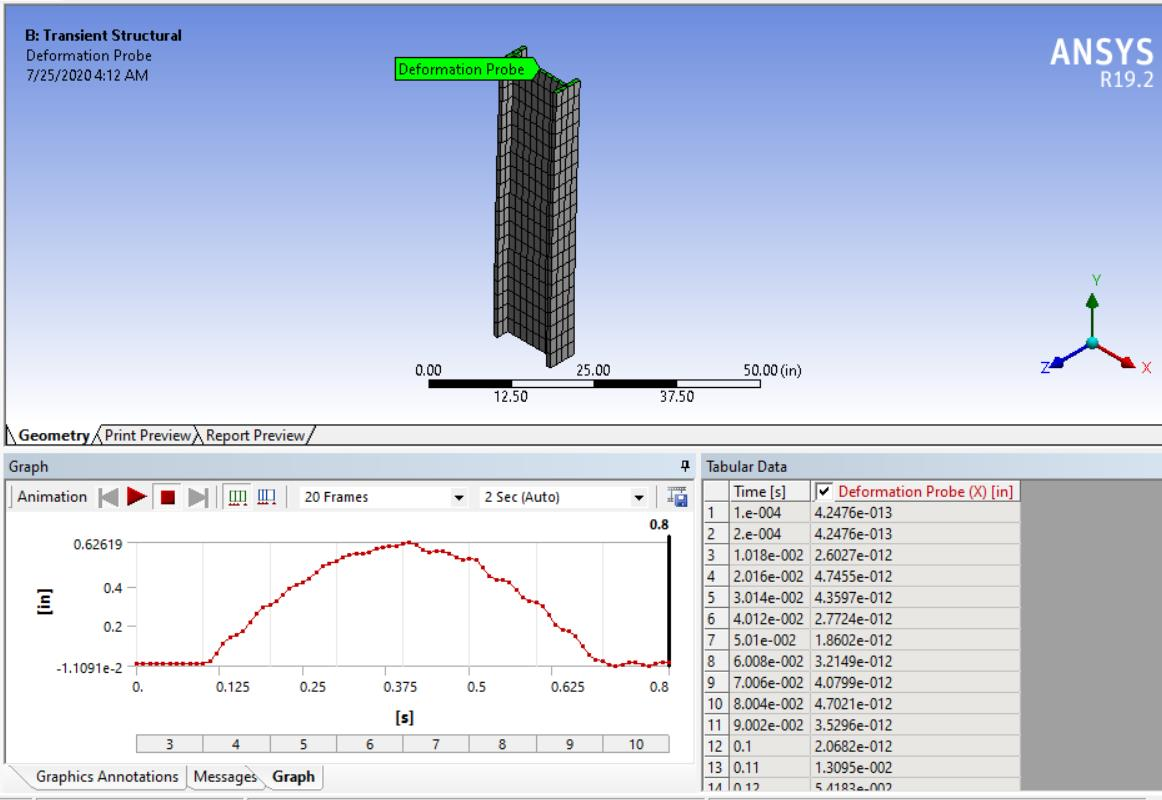the correct displacement should be as follows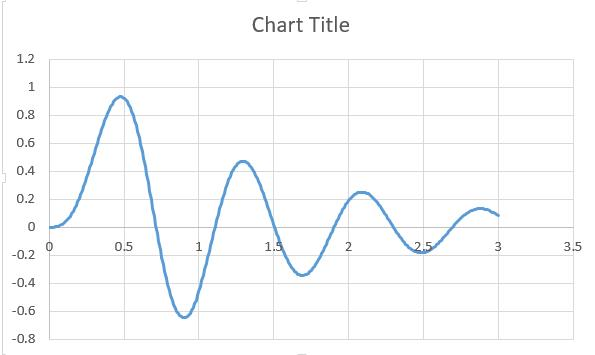• Any dynamics problem should have a Modal analysis done first.

What are the first six natural frequencies of this beam with the point mass on it?

Which of those modes has vibration in the X direction?

• i want to conduct a direct integration time history analysis without linking modal analysis solution as i am planning to do a non linear problem and i think i read in mechanical help that both methods "with or without linking modal analysis solution" can be done

• I understand what you want to do. Yes, you can do transient structural with or without linking to a modal analysis but to do any nonlinear computation, the setup must not be linked to a Modal analysis solution.

You should understand that a Modal analysis provides valuable information that you need in order to setup the direct integration of the full model, without linking to the modal. If you want further help from me, you will answer the following two questions.

What are the first six modal frequencies of this beam with the point mass on it?

Which of those modes has vibration in the X direction?

• thanks Peter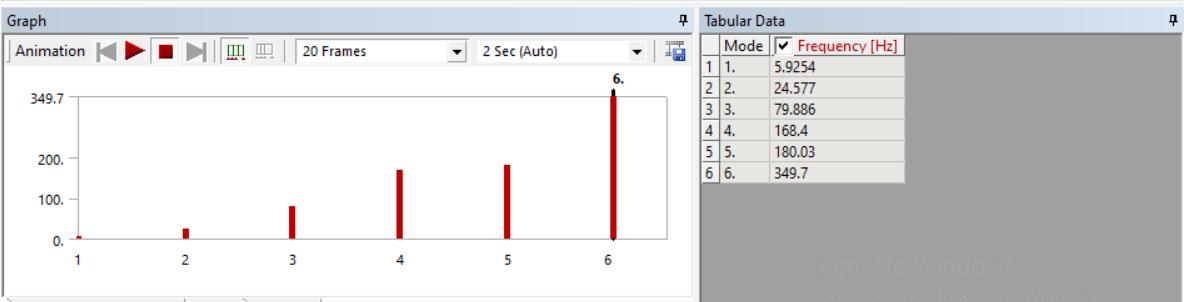and the mode that has vibration in x direction is mode 2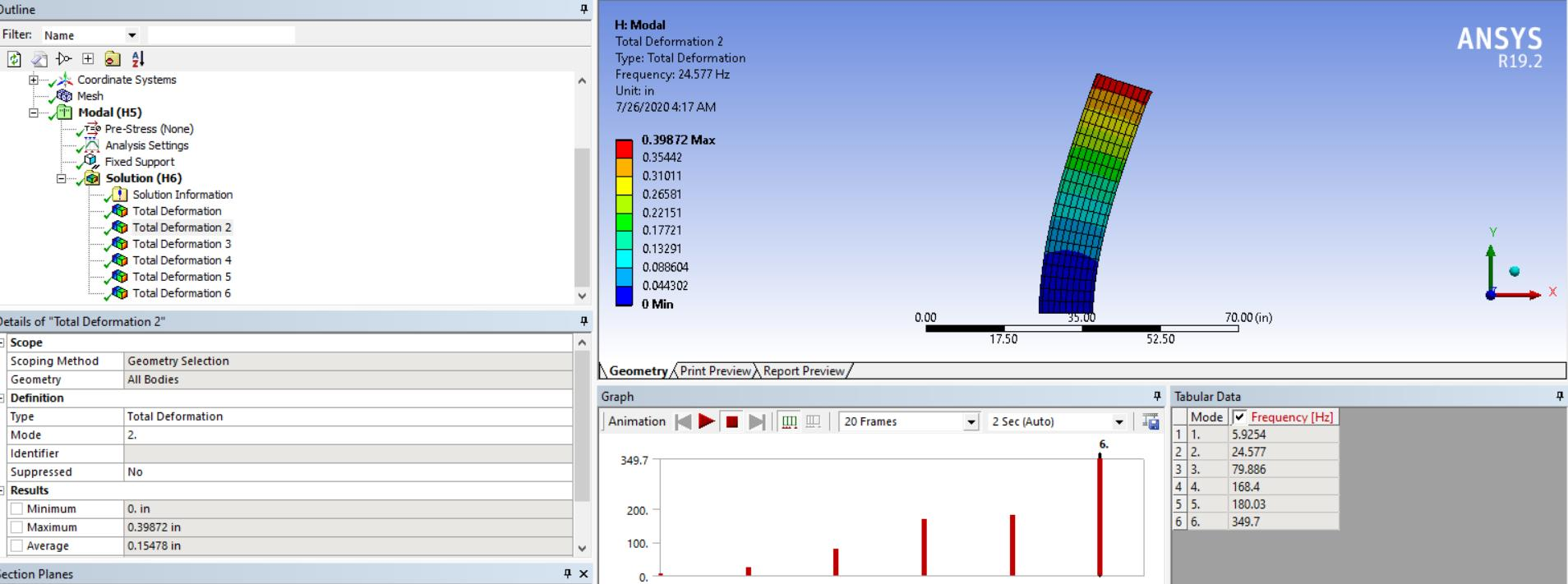• The Modal shows that the structure vibrates in the X direction at about 24 Hz.

The period of a 24 Hz frequency is 1/24 seconds = 0.042 s

To excite that vibration mode, a pulse of half that period is needed or a pulse that lasts no more than 0.021 s.

Now compare that length of time with the half sine shape you show for steps 3-9 above. That pulse lasts 0.6 s.

Can you see that the pulse you applied is 28 times too long?  Can you see how important a Modal analysis is?

Get rid of the two initial time steps; zero velocity is the default condition, you don't do anything special to get that.

If you want the first step to compute the static deformation due to gravity, turn off Time Integration and let Step End Time be 1e-5 s.

Use a pulse that has a duration of about 0.02 seconds. You can do this in Step 2, you don't need more steps.

Let Step 2 have an End time of 0.21 s and you will see 5 cycles in the transient simulation.
Let the Initial Time Increment be 1e-3 s
Let the Minimum Time Increment be 1e-4 s
Let the Maximum Time Increment be 0.002 s and you will get at least 100 data points along the time history.

In the Force definition, use Tabular input. You can use as many rows as you like if you want to make your half sine pulse shape.
Do not type this in by hand, type it into Excel, copy then Paste it into the Force table. Make sure the direction is set to point along X.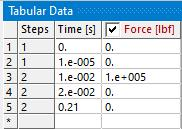Note that exciting Mode 2 only is difficult and some motion in the Mode 1 Z direction can leak into the results.